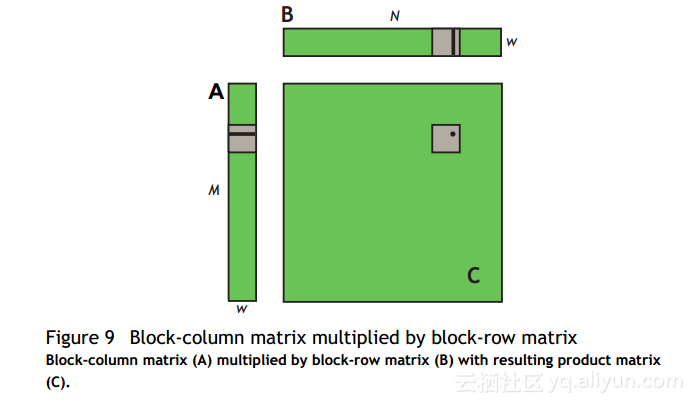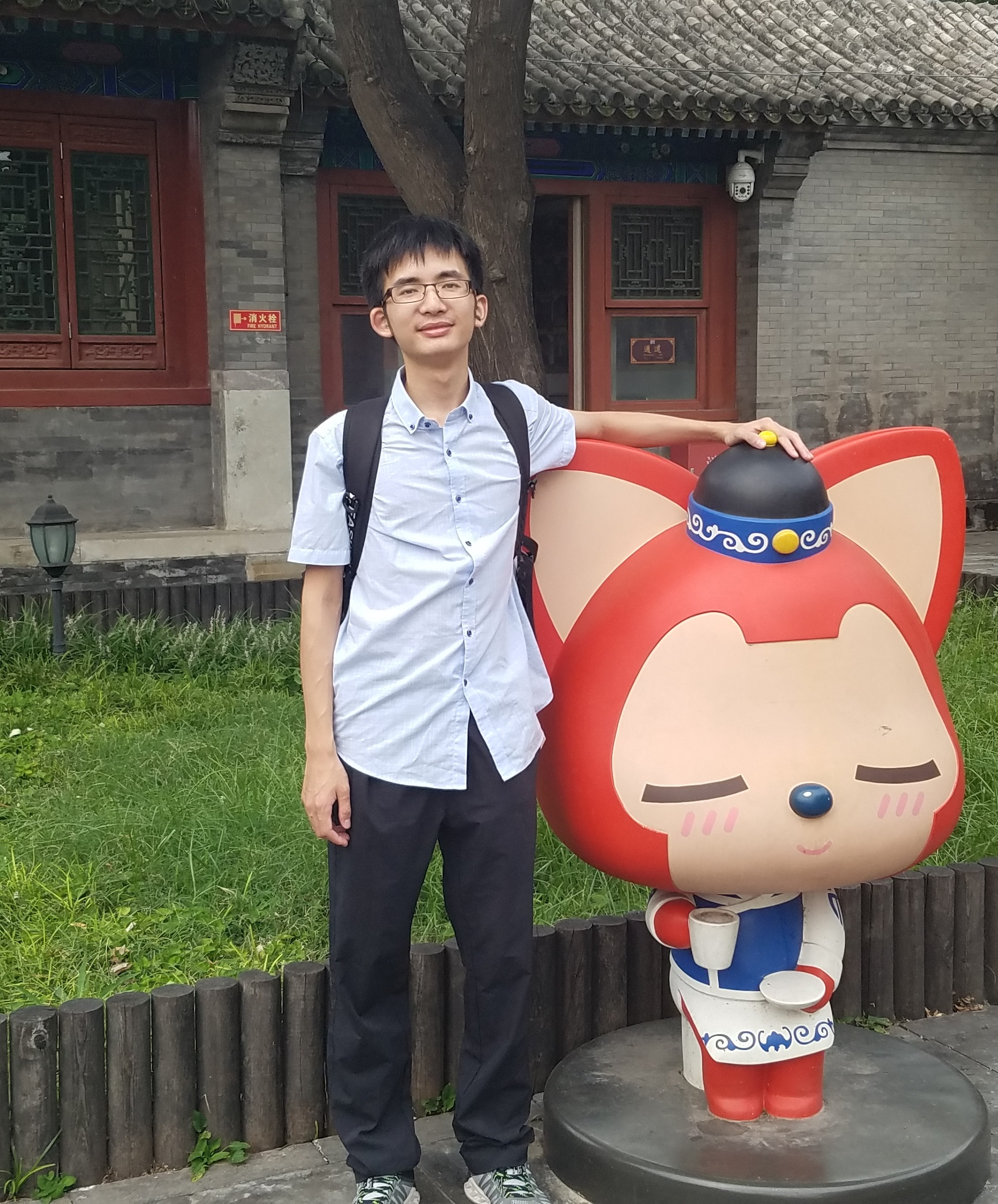# CUDA实践指南（十六）

+关注继续查看__global__ void simpleMultiply(float *a, float* b, float *c,
int N)
{
int row = blockIdx.y * blockDim.y + threadIdx.y;
int col = blockIdx.x * blockDim.x + threadIdx.x;
float sum = 0.0f;
for (int i = 0; i < TILE_DIM; i++) {
sum += a[row*TILE_DIM + i] * b[i*N + col];
}
c[row*N + col] = sum;
}

C.行和列是C中元素的行和列由特定的线程计算。 for循环结束我将一行A乘以B的列，然后写入C.CUDA实践指南（十七）
966 0CUDA实践指南（十五）
1053 0CUDA实践指南（十四）
1046 0CUDA实践指南（十二）
1259 0CUDA实践指南（二十三）
967 0CUDA实践指南（十一）
1229 0CUDA实践指南（二十二）
2173 0CUDA实践指南（二十六）
2317 0CUDA实践指南（二十一）
1080 0CUDA实践指南（二十五）
2329 0
night李12125

Python基础快速入门实战教程37552832332

TensorFlow基础教程384631

Tensorflow2.0入门与实战28919

Pytorch实战773729